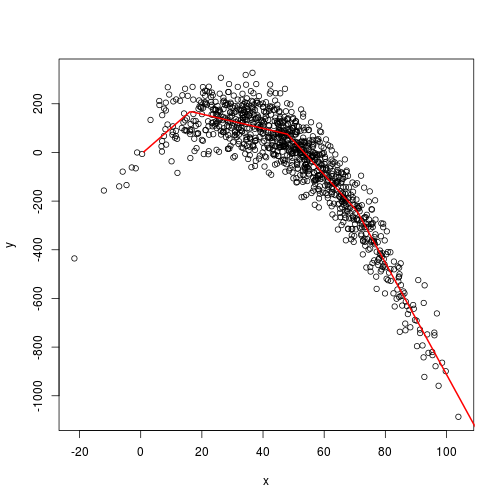## Paul Johnson <pauljohn@ku.edu>
## 2014-05-28
## MARS: Multivariate Adaptive Regression Splines

## I found it a bit difficult to interact with the
## MARS fitted objects created by the functions in
## the mda package.  Later, I found a very nice
## package "earth" with an implementation of MARS

## As far as I can see, estimates from earth are
## same, but the follow up methods and summary
## printout are much better.

library(earth)

## Loading required package: plotmo

x <- rnorm(1000, 50, 20)
y <- 11.2*x -.21*x^2 + rnorm(1000, m = 0, s = 79)
df <- data.frame(x,y)
m3 <- earth(y ~ x, dat = df)
summary(m3)

## Call: earth(formula=y~x, data=df)
##
##              coefficients
## (Intercept)        516.90
## h(x-16.2033)       -13.90
## h(47.9672-x)       -10.96
## h(x-70.7611)        -9.05
##
## Selected 4 of 5 terms, and 1 of 1 predictors
## Importance: x
## Number of terms at each degree of interaction: 1 3 (additive model)
## GCV 5656    RSS 5577445    GRSq 0.8915    RSq 0.8928

plot(y ~ x, data = df)
xnew = seq_along(x)
ppp <- predict(m3, newdata = data.frame(x = xnew))
lines(ppp ~ xnew, data = df, col = "red", lwd = 2)m3 <- earth(y ~ x, dat = df, degree = 3, trace = 1)

## x is a 1000 by 1 matrix: 1=x
## y is a 1000 by 1 matrix: 1=y
## Forward pass term 1, 2, 4, 6, 8
## Reached delta RSq threshold (DeltaRSq 0.00048 < 0.001) at 7 terms
## After forward pass GRSq 0.891 RSq 0.893
## Prune method "backward" penalty 3 nprune 5: selected 4 of 5 terms, and 1 of 1 predictors
## After backward pass GRSq 0.891 RSq 0.893

summary(m3)

## Call: earth(formula=y~x, data=df, trace=1, degree=3)
##
##              coefficients
## (Intercept)        516.90
## h(x-16.2033)       -13.90
## h(47.9672-x)       -10.96
## h(x-70.7611)        -9.05
##
## Selected 4 of 5 terms, and 1 of 1 predictors
## Importance: x
## Number of terms at each degree of interaction: 1 3 (additive model)
## GCV 5673    RSS 5577445    GRSq 0.8912    RSq 0.8928

plot(y ~ x, data = df)
xnew = seq_along(x)
ppp <- predict(m3, newdata = data.frame(x = xnew))
lines(ppp ~ xnew, data = df, col = "red", lwd = 2)